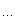# 魔のkyo的工作室

posts - 225, comments - 62, trackbacks - 0, articles - 0
:: 首页 :: 新随笔 :: 联系 :: 聚合:: 管理

1 #include <iostream>
2 #include <cstdio>
3 using namespace std;
4
5 template <typenameArgs> // 通过typename声明的Args称为模板参数包，注意是写在typename后面的
6 void func(const Args&args) // 这里的const Args&称为函数参数类型包，而声明出的args称为函数参数包
7 {
8     printf(args); // 这里的args称为对函数参数包的展开
9 }
10
11 int main(void)
12 {
13     func("%s %d""Hello world"123);
14 }
15

Hello world 123

1 #include <iostream>
2 #include <cstdio>
3 using namespace std;
4
5 // 递归终止
6 void func()
7 {
8
9 }
10
11 template <typename T, typenameArgs>
12 void func(const T& obj, const Args&args)
13 {
14     cout << obj << " ";
15     func(args); // 递归调用
16 }
17
18 int main(void)
19 {
20     func("Hello world"123); //因为改成通过cin，前面的格式化字符串删掉了
21 }

1 #include <iostream>
2 #include <cstdio>
3 using namespace std;
4
5 template <typename T, typenameArgs>
6 void func(const T& obj, const Args&args)
7 {
8     cout << obj << " ";
9     if (sizeof(Args) > 0) {
10         func(args); // 递归调用
11     }
12 }
13
14 int main(void)
15 {
16     func("Hello world"123); //因为改成通过cin，前面的格式化字符串删掉了
17 }
18

1 #include <iostream>
2 #include <cstdio>
3 using namespace std;
4
5 template <typename T, typenameArgs>
6 void func(const T& obj, const Args&args);
7
8 // 声明一个带有一个bool参数的模板struct
9 template<bool Flag>
10 struct If_Func;
11
12 // 下面对bool参数分别进行true/false两种特化实现，以此实现编译期的分支
13 template<>
14 struct If_Func<true>
15 {
16     template<typenameArgs>
17     static void call (const Args&args) {
18         func(args);
19     }
20 };
21
22 template<>
23 struct If_Func<false>
24 {
25     template<typenameArgs>
26     static void call(const Args&args) {
27
28     }
29 };
30
31 template <typename T, typenameArgs>
32 void func(const T& obj, const Args&args)
33 {
34     cout << obj << " ";
35     constexpr bool flag = sizeof(Args) > 0;
36     If_Func<flag>::call(args);
37 }
38
39 int main(void)
40 {
41     func("Hello world"123); //因为改成通过cin，前面的格式化字符串删掉了
42 }

#include <iostream>
#include
<tuple>
using namespace std;

int main(void)
{
tuple
<intdoublestring> t = make_tuple(13.14"Hello world");
cout
<< typeid(t).name() << endl;
cout
<< std::tuple_size<decltype(t)>::value << endl; // 输出tuple的元素个数
cout << std::get<0>(t) << endl; // 输出tuple的第0个元素
cout << std::get<1>(t) << endl; // 输出tuple的第1个元素
cout << std::get<2>(t) << endl; // 输出tuple的第2个元素
}

template<typenameArgs>
class my_tuple;

template
<>
class my_tuple<>
{

};

template
<typename T, typenameArgs>
class my_tuple<T, Args> : public my_tuple<Args>
{
public:

protected:
T _obj;
};

cout << make_tuple(123, 3.14, "Hello") << endl;

print( (123, 3.14, "Hello") )

template <typename... Args>
ostream& operator << (ostream& out, const tuple<Args...> t);

#include <iostream>
#include
<string>
#include
<tuple>
#include
<sstream>
using namespace std;

template
<size_t N>
struct PrintHelper;

template
<>
struct PrintHelper<1>
{
template
<typenameArgs>

static void recursive_print(ostream& outconst tuple<Args> t)
{

out << "(" << std::get<0>(t) << "";
}
};

template
<size_t N>
struct PrintHelper
{
template
<typenameArgs>

static void recursive_print(ostream& outconst tuple<Args> t)
{
PrintHelper
<- 1>::recursive_print(out, t);

out << std::get<- 1>(t) << "";
}

template
<typenameArgs>

static void print(ostream& outconst tuple<Args> t)
{
PrintHelper
<- 1>::recursive_print(out, t);

out << std::get<- 1>(t) << ")";
}
};

template
<typenameArgs>
ostream
& operator << (ostream& outconst tuple<Args> t)
{
PrintHelper
<tuple_size<decltype(t)>::value >::print(out, t);

// PrintHelper<sizeof(Args)>::print(out, t); // 和上面等价
return out;
}

int main(void)
{
cout
<< std::make_tuple(1233.14"Hello"<< endl;
}

(123, 3.14, Hello)
Hello的两端并没有引号，如果想加上引号，可以再次利用模板元编程技巧通过编译时的分支把string类型的输出方法特化，此处不再给出具体代码，留给读者自行探索。